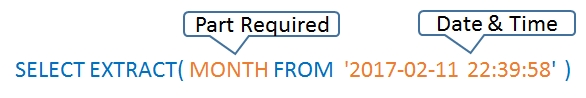# EXTRACT() : Parts of Date & Time in MySQL``SELECT EXTRACT(YEAR FROM '2017-03-08');``
Output is 2017
Input format must be in "YYYY-MM-DD HH-MM-SS" , we can use STR_TO_DATE() for any other format ( See examples below )
``SELECT EXTRACT( DAY FROM  '2017-04-28' );   ``
Output 28
``SELECT EXTRACT( DAY FROM  '2017-04-28' );   ``
Output 28
`SELECT EXTRACT( MONTH FROM  '2017-10-10' );`
Output is 10
`SELECT EXTRACT( YEAR_MONTH FROM  '2017-03-08' )`
Output is 201703

## EXTRACT() with STR_TO_DATE()

We can use other type input date formats also.
`SELECT EXTRACT( YEAR FROM STR_TO_DATE('5-07-2017', '%d-%m-%Y') )`

## EXTRACT() with NOW()

`SELECT EXTRACT( YEAR FROM NOW() )`
Output is Present Year 2017
`SELECT EXTRACT( HOUR FROM NOW( ) )`
Output is 04
``SELECT EXTRACT( MINUTE FROM NOW( ) )``
Output is 45
``SELECT EXTRACT( SECOND FROM NOW( ) )``
Output is 09
``SELECT EXTRACT( MICROSECOND FROM '2017-02-11 22:39:59.000020' )``
Output is 20
``SELECT EXTRACT( HOUR_MINUTE FROM NOW( ) )``
Output is 0445
``SELECT EXTRACT( MINUTE_SECOND FROM NOW( ) )``
Output is 4509
``SELECT EXTRACT( SECOND_MICROSECOND FROM '2017-02-11 22:39:59.000020' ) //Output 59000020``

## EXTRACT() with LOCALTIMESTAMP()

``SELECT EXTRACT( YEAR FROM localtimestamp() )``
``SELECT EXTRACT( MONTH FROM localtimestamp() )``
``SELECT EXTRACT( HOUR_MINUTE FROM localtimestamp() )``

## EXTRACT() with CURDATE()

``SELECT EXTRACT( DAY FROM CURDATE() )``
Output is 25
``SELECT EXTRACT( MONTH FROM CURDATE() )``
Output is 03
`SELECT EXTRACT( YEAR FROM CURDATE() )`
Output is 2023
Subscribe to our YouTube Channel here

## Subscribe

* indicates required
Subscribe to plus2netplus2net.com

Post your comments , suggestion , error , requirements etc here .